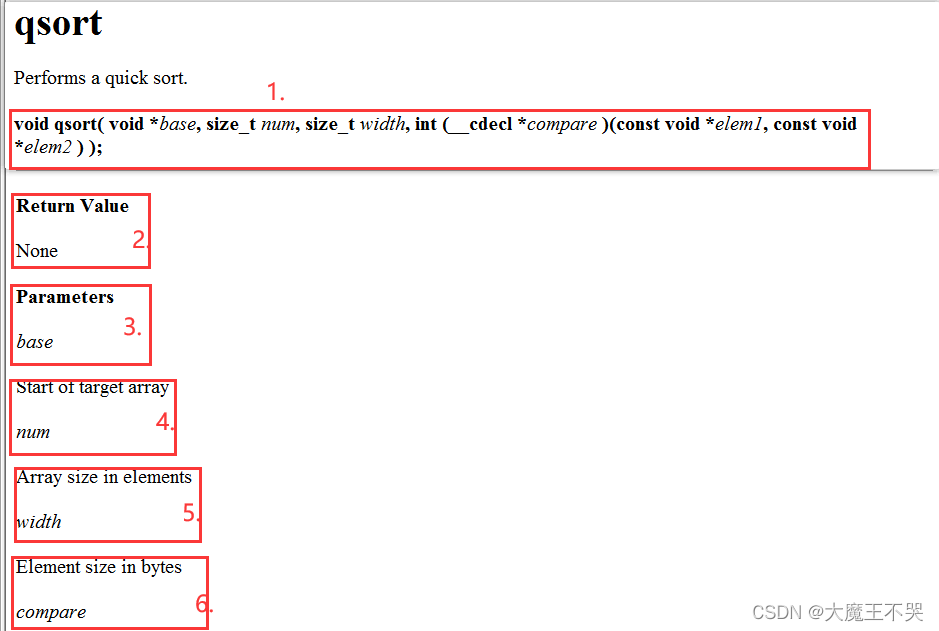﻿ 你真的理解C语言qsort函数吗 带你深度剖析qsort函数_C 语言_脚本之家
C 语言# 你真的理解C语言qsort函数吗 带你深度剖析qsort函数

## 二、简单冒泡排序法

```#include <stdio.h>
int main()
{
int arr = { 9,8,7,6,5,4,3,2,1,0 };
int i = 0;
int sz = sizeof(arr);
for (i = 0; i < sz-1; i++)
{
int j = 0;
for (j = 0; j < sz-1-i; j++)
{
int tep = 0;
if (arr[j] > arr[j + 1])
{
tep = arr[j];
arr[j] = arr[j + 1];
arr[j + 1] = tep;
}
}
}
return 0;
}```

## 三、qsort函数的使用

### 1、qsort函数的介绍### 2、qsort函数的运用

#### 2.1、qsort函数排序整型数组

```#include<stdio.h>
#include <stdlib.h>
int cmp_int(const void* e1, const void* e2)
{
return *(int*)e1 - *(int*)e2;
}
int main()
{
int arr = { 9,8,7,6,5,4,3,2,1,0 };
int sz = sizeof(arr) / sizeof(arr);
qsort(arr, sz, sizeof(arr), cmp_int);
int i = 0;
for(i = 0;i<sz;i++)
printf("%d ", arr[i]);
}```1.qsort第四个参数（函数地址）为什么不需要传参？

2.为什么函数定义里面的参数是空指针类型，而不是特定类型？

3.空指针是什么意思，怎么用？

4.为什么com_int函数在用的时候要把参数强转为int？

#### 2.2、qsort函数排序结构体

```#include <stdio.h>
#include <stdlib.h>
#include <string.h>
struct stu
{
char name;
int age;
};
//排序结构体年龄的回调函数
int cmp_struct_stu_age(const void*e1,const void*e2)
{
return ((struct stu*)e1)->age - ((struct stu*)e2)->age;
}
//排序结构体姓名的回调函数
int cmp_struct_stu_name(const void* e1, const void* e2)
{
return strcmp(((struct stu*)e1)->name, ((struct stu*)e1)->name);
}
//运用qsort函数排序结构体年龄
void test1()
{
struct stu s = { {"xiaoming",30},{"lihua",60},{"wangli",40} };
int sz = sizeof(s) / sizeof(s);
qsort(s, sz, sizeof(s), cmp_struct_stu_age);
}
//运用qsort函数排序结构体名字
void test2()
{
struct stu s = { {"xiaoming",30},{"lihua",60},{"wangli",40} };
int sz = sizeof(s) / sizeof(s);
qsort(s, sz, sizeof(s), cmp_struct_stu_name);
}
int main()
{
test1();
//test2();
return 0;
}
```

## 四、利用冒泡排序模拟实现qsort函数

```#include <stdio.h>
int cmp_int(const void* e1, const void* e2)
{
return *(int*)e1 - *(int*)e2;
}
void swap(char* buf1, char* buf2, int width)
{
int i = 0;
for (i = 0; i < width; i++, buf1++, buf2++)
{
char tep = 0;
tep = *buf1;
*buf1 = *buf2;
*buf2 = tep;
}
}
void my_qsort(const void* base, int sz, int width, int(*cmp)(const void* e1, const void* e2))
{
int i = 0;
for (i = 0; i < sz - 1; i++)
{
int j = 0;
for (j = 0; j < sz - 1 - i; j++)
{
if(cmp((char*)base+j*width,(char*)base+(j+1)*width)>0)
swap((char*)base + j * width, (char*)base + (j + 1) * width,width);
}
}
}
void test3()
{
int arr = { 9,8,7,6,5,4,3,2,1,0 };
int sz = sizeof(arr) / sizeof(arr);
my_qsort(arr, sz, sizeof(arr), cmp_int);
int i = 0;
for (i = 0; i < sz; i++)
printf("%d ", arr[i]);
}
int main()
{
test3();
return 0;
}```

1.在my_qsort函数中，我们在比较函数（cmp）中传入的是内容解释：
☃️(char*)base+jwidth 这个实际上就是原始的base是空指针类型，所以我们无法直接使用，但我们也不确定传来的具体是什么类型，因此我们先将其强制类型转换为char，然后根据指针加几就跳过几个步长个字节来确定每次跳过一个元素。
2.在my_qsort函数中，我们的swap函数也是一个重要部分，它是如何实现的呢？
☃️首先和1刚开始一样，我们传的实参的形式就是为了保证每次可以跳过一个元素，然后当传到swap函数里后，我们不像cmp函数一样有提前准备好的强转类型，因此我们需要用一种特别的方法保证可以互换元素，那么我们会想到元素是怎样储存的呢，是以二进制以字节为单位储存的，那么我们如果需要交换元素，是不是只需要把每个字节都交换一次就好了呢？答案是肯定的，于是我们也将传来的地址强制类型转换为char*，保证每次交换的是一个字节，然后又有一个问题就是我们需要交换几次呢，还记得之前传的那个参数width吗，它是每个元素的宽度，单位是字节，因此我们可以通过一个for循环，修女换width次来使得一个元素所占的字节全部交换。

```#include <stdio.h>
#include <stdlib.h>
#include <string.h>
//排序整型数组顺序时的回调函数
int cmp_int(const void* e1, const void* e2)
{
return *(int*)e1 - *(int*)e2;
}
//声明结构体类型
struct stu
{
char name;
int age;
};
//排序结构体年龄顺序时的回调函数
int cmp_struct_stu_age(const void*e1,const void*e2)
{
return ((struct stu*)e1)->age - ((struct stu*)e2)->age;
}
//排序结构体名字顺序时的回调函数
int cmp_struct_stu_name(const void* e1, const void* e2)
{
return strcmp(((struct stu*)e1)->name, ((struct stu*)e1)->name);
}
//运用qsort函数排序整型数组
void test1()
{
int arr = { 9,8,7,6,5,4,3,2,1,0 };
int sz = sizeof(arr) / sizeof(arr);
qsort(arr, sz, sizeof(arr), cmp_int);
}
//运用qsort函数排序结构体
void test2()
{
struct stu s = { {"xiaoming",30},{"lihua",60},{"wangli",40} };
int sz = sizeof(s) / sizeof(s);
qsort(s, sz, sizeof(s), cmp_struct_stu_name);
//qsort(s, sz, sizeof(s), cmp_struct_stu_age);
}
//模拟qsort中的交换函数
void swap(char* buf1, char* buf2, int width)
{
int i = 0;
for (i = 0; i < width; i++, buf1++, buf2++)
{
char tep = 0;
tep = *buf1;
*buf1 = *buf2;
*buf2 = tep;
}
}
//模拟qsort
void my_qsort(const void* base, int sz, int width, int(*cmp)(const void* e1, const void* e2))
{
int i = 0;
for (i = 0; i < sz - 1; i++)
{
int j = 0;
for (j = 0; j < sz - 1 - i; j++)
{
if(cmp((char*)base+j*width,(char*)base+(j+1)*width)>0)
swap((char*)base + j * width, (char*)base + (j + 1) * width,width);
}
}
}
//运用模拟qsort函数(my_qsort)排序整型数组
void test3()
{
int arr = { 9,8,7,6,5,4,3,2,1,0 };
int sz = sizeof(arr) / sizeof(arr);
my_qsort(arr, sz, sizeof(arr), cmp_int);
int i = 0;
for (i = 0; i < sz; i++)
printf("%d ", arr[i]);
}
//运用模拟qsort函数(my_qsort)排序结构体
void test4()
{
struct stu s = { {"xiaoming",30},{"lihua",60},{"wangli",40} };
int sz = sizeof(s) / sizeof(s);
my_qsort(s, sz, sizeof(s), cmp_struct_stu_name);
//qsort(s, sz, sizeof(s), cmp_struct_stu_age);
}
int main()
{
//test1();  //用qsort实现整型数组排序
//test2();  //用qsort实现结构体排序
test3();    //用模拟qsort函数实现整型数组排序
//test(4)   //用模拟qsort函数实现结构体排序
return 0;
}```